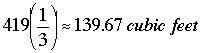SEARCH HOMEMath Central Quandaries & QueriesQuestion from Kathy: We are needing to dispose of concrete that we are tearing out of our backyard and I need to figure the dimensions and weight. I figured we have approx. 419 sq ft of concrete at 4" thick. Could you tell me the how much that comes to in cubic ft, cubic yards and weight. So I can get some estimates on pricing to haul off and dispose of. thank youHello Kathy,

Since you have already determined the area covered by concrete we need only to multiply by the thickness to obtain the volume of concrete.  To do so we must use the same units of measure, namely feet.   Since four inches is a third of a foot we get:You can use Google to convert that into cubic yards, or some other unit of measure.

(Type 139.67 cubic feet to cubic yards into Google.)

Determining the weight of this volume of concrete requires knowing the density of the concrete.  The density may change depending on the purpose of the concrete.  If you can find out what the density of your concrete is, then finding the weight comes down to multiplying that number by the volume.

Tyler

Kathy,

You can estimate the density of concrete by looking in the SIMetric site. This site uses the units of kilograms per cubic meter but again you can use Google to convert your 139.67 cubic feet to cubic meters, multiply by the density to obtain the weight in kilograms and then use Google again to convert the weight to pounds.

HarleyMath Central is supported by the University of Regina and The Pacific Institute for the Mathematical Sciences.